# Chip model for multiplication

This is the second part of a series of posts showing how to visually represent the four operations of whole numbers using the CHIP MODEL. The view the first part in which we show how to model addition and subtraction, go here.

Just as the chip model is a particularly effective way in visually represent addition and subtraction, we can also visually model multiplication with the chip model.

There are a variety to think of multiplication such as skip counting, area model, and repeated addition. With the chip model, however, we will rely on thinking of multiplication as “equal groups”.

34 x 7 means “34 groups of 7” and also “7 groups of 34”.

Most people would think it is easier to model 34 x 7 as “7 groups of 34”.

 We begin by modeling 34 on the place value chart.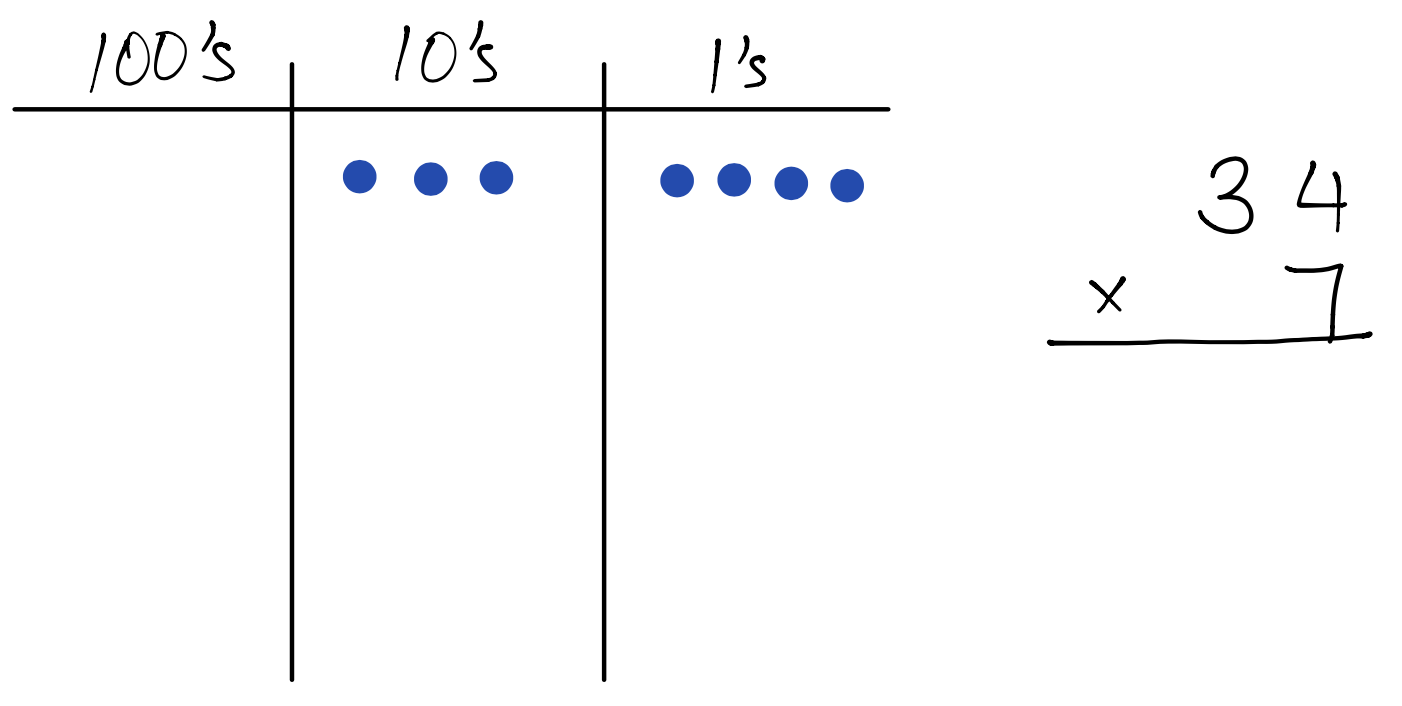Then we model seven groups of 34. At this point, we can see that the unsimplified product is 21 tens and 28 ones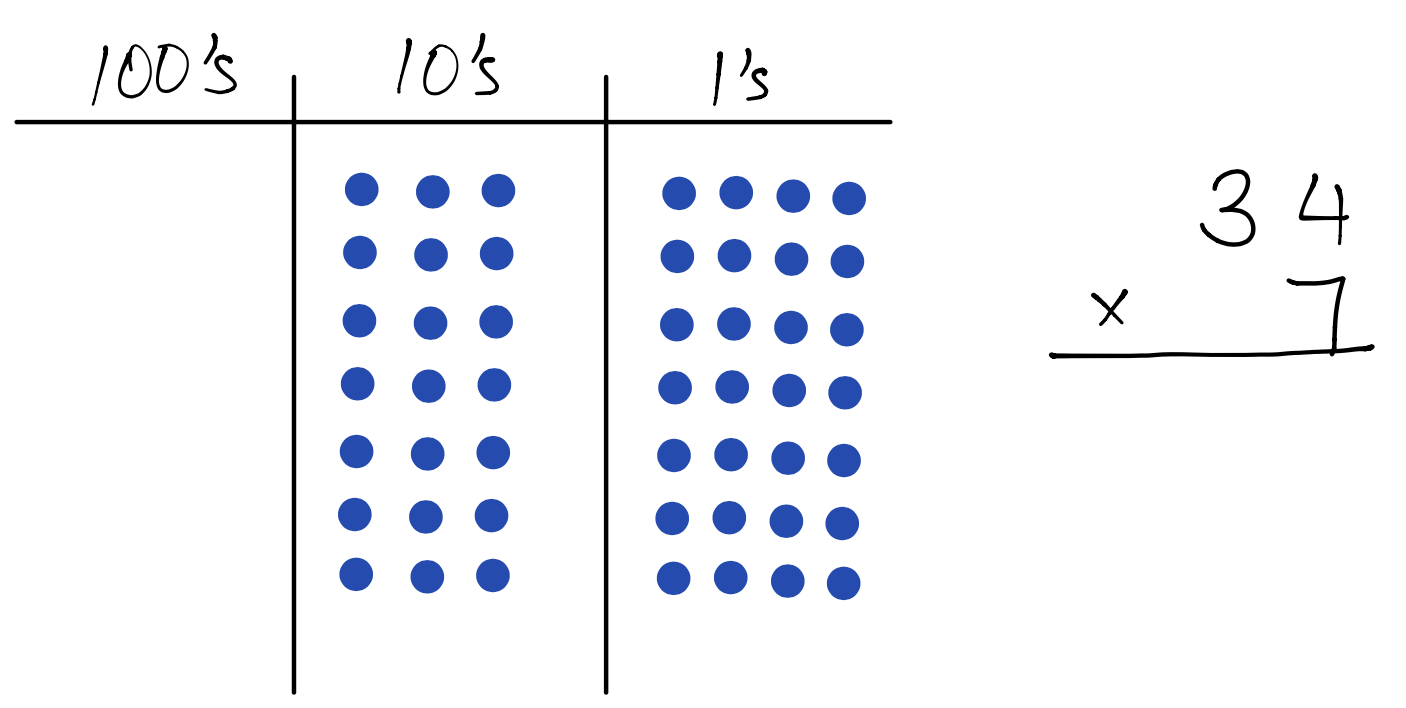Now we look for opportunities to do the exchange rate of 10 littles equals 1 big. We see there is a multiple opportunities to do so because there are more than 10 dots in both the tens column and the ones column. It does not matter which exchanges we do first.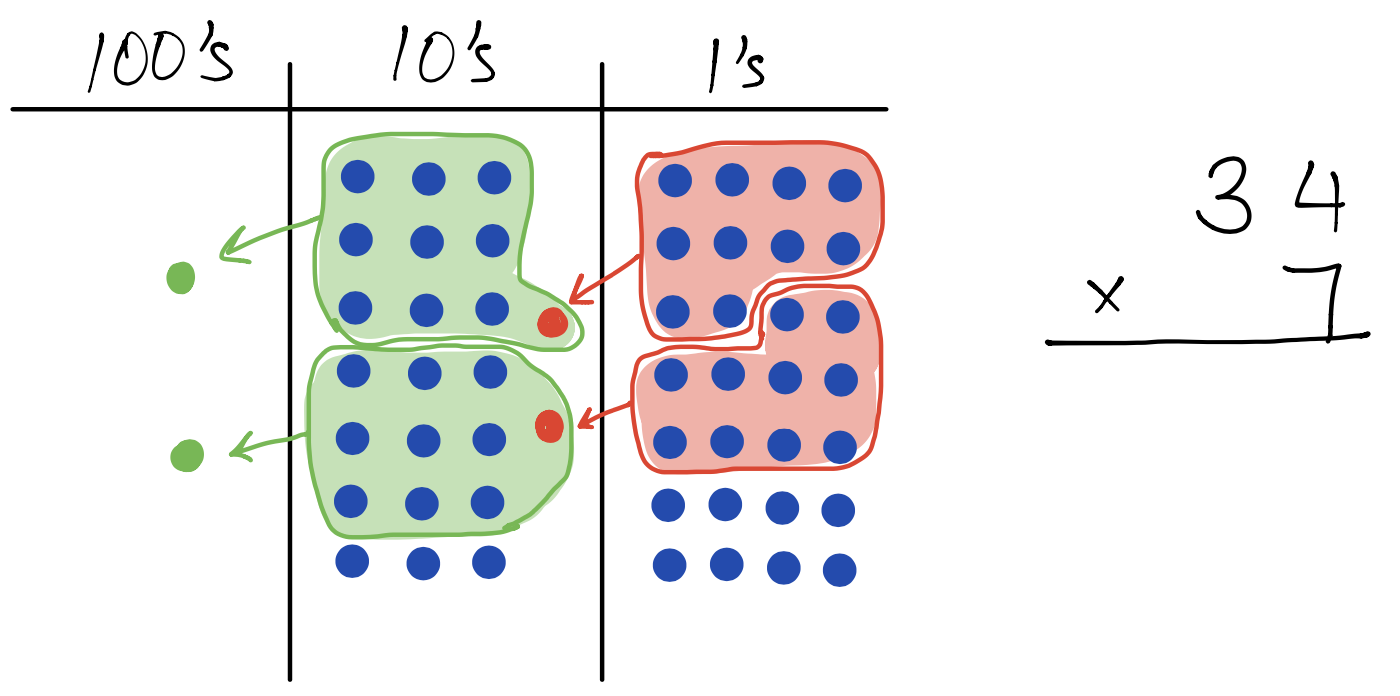After completing all the possible exchanges of 10 littles equals 1 big, we count the remaining dots in each column. 2 hundreds and 3 tens and 8 ones The product is 238.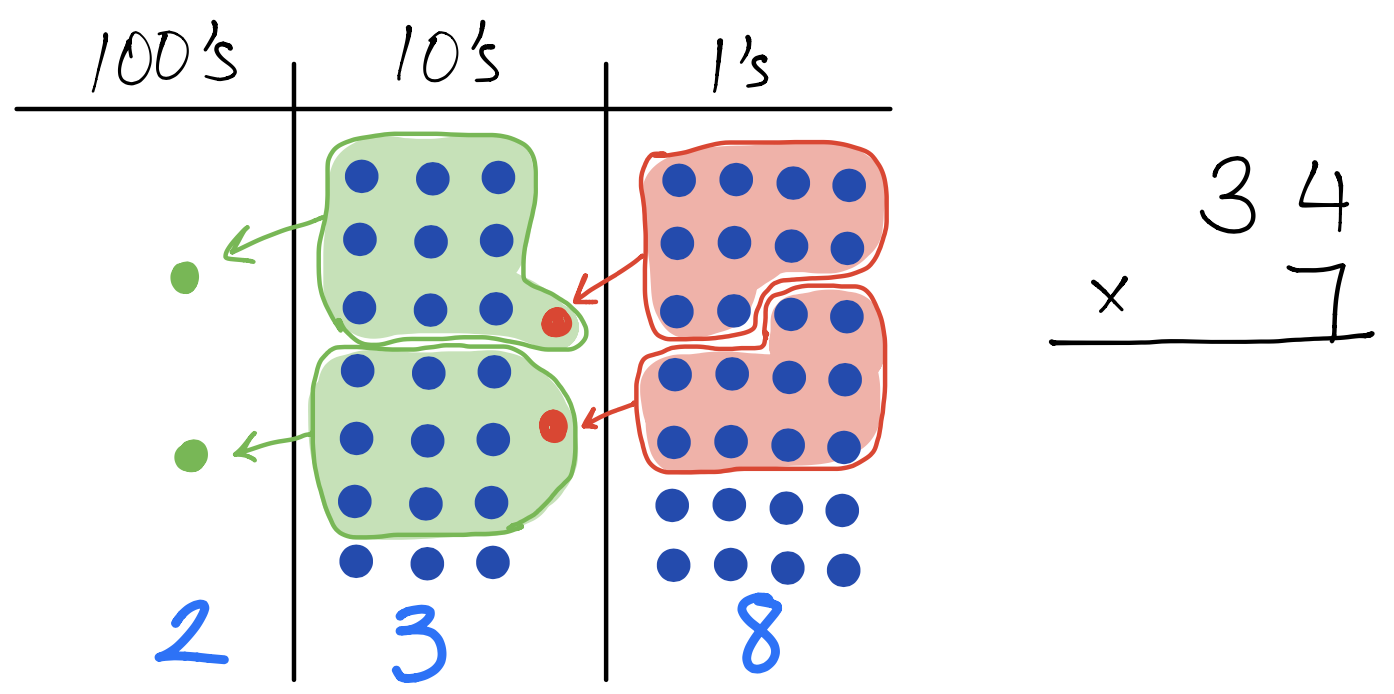Let’s do a slightly larger example of a 3-digit number multiplied by a 1-digit number.

137 x 5 will be modeled as “5 groups of 137”

 We begin by modeling 137 on the place value chart.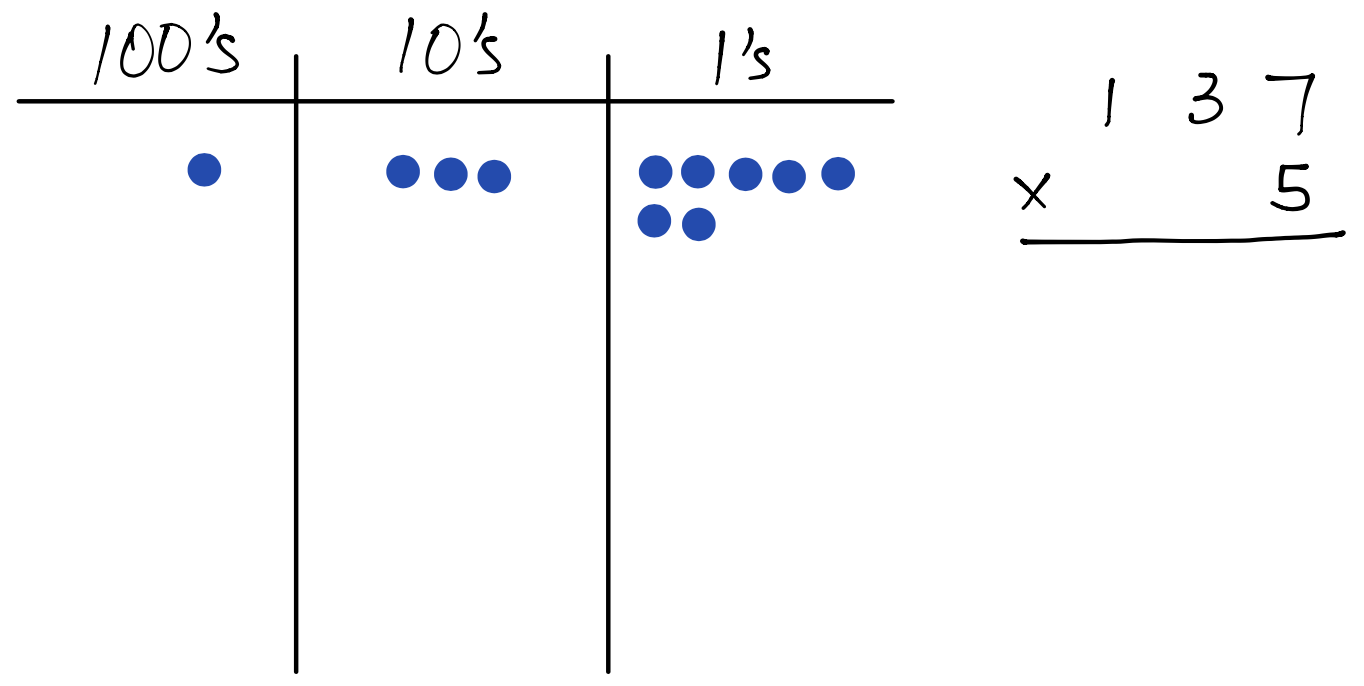Then we model five groups of 137. At this point, we can see that the unsimplified product is 5 hundreds and 15 tens and 35 ones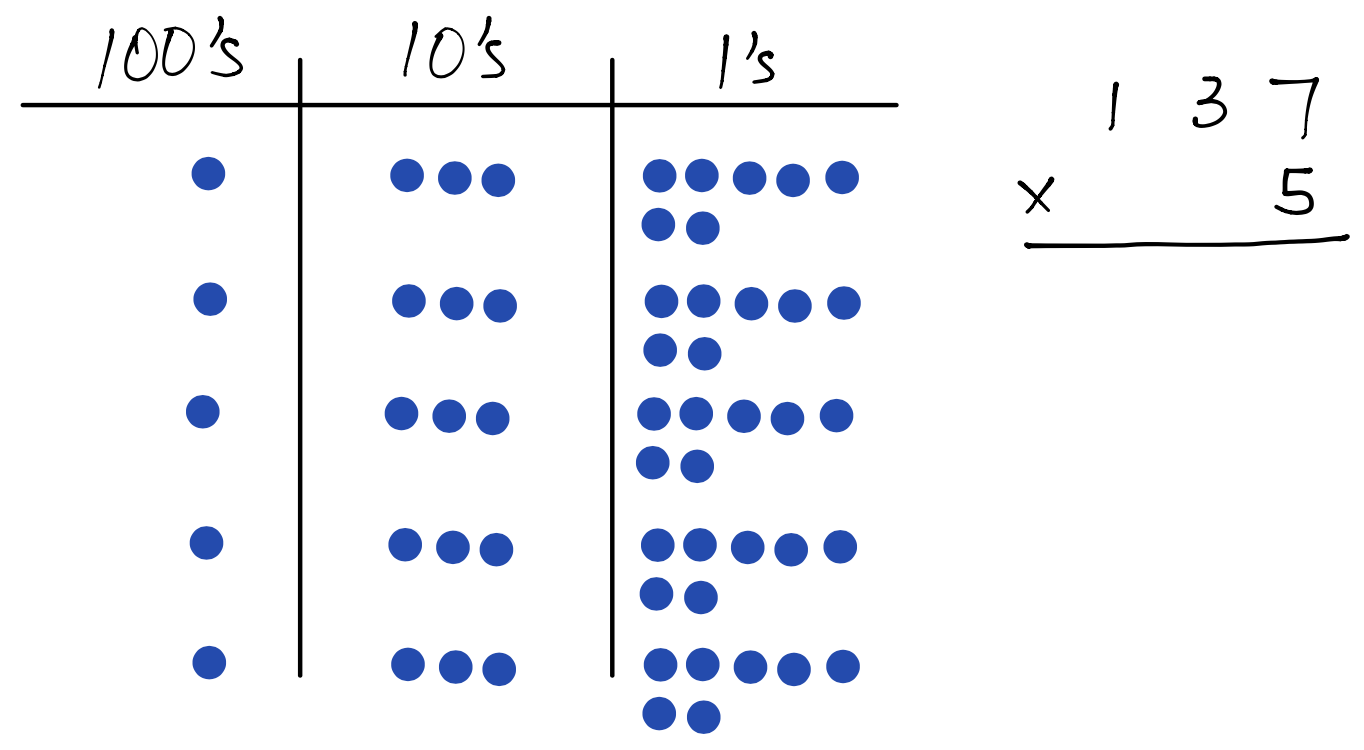Now we look for opportunities to do the exchange rate of 10 littles equals 1 big. We see there is a four opportunities to do so.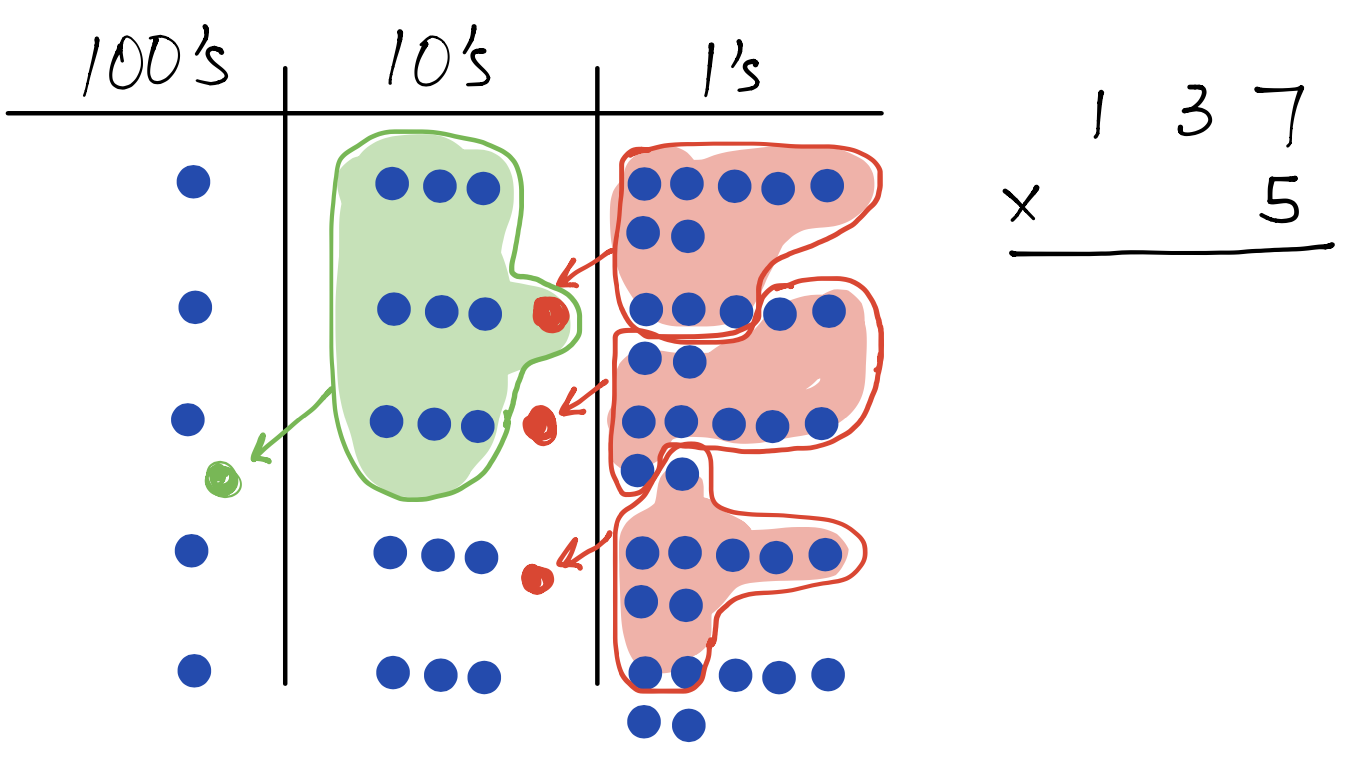After completing all the possible exchanges of 10 littles equals 1 big, we count the remaining dots in each column. 6 hundreds and 8 tens and 5 ones The product is 685.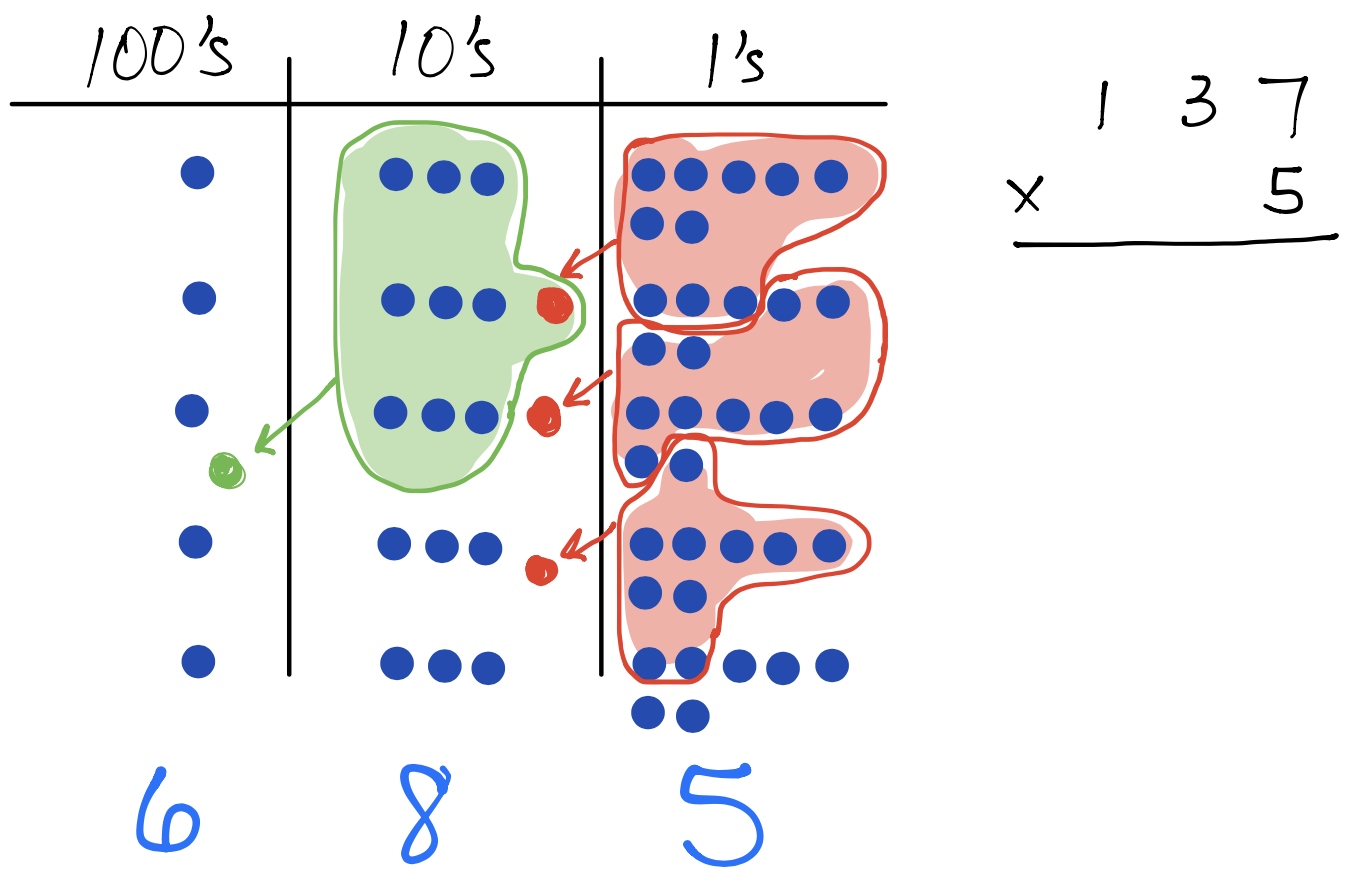Eventually, students will show the chip model and the algorithm side-by-side, using the chip model one the left and recording each step with numbers on the right side.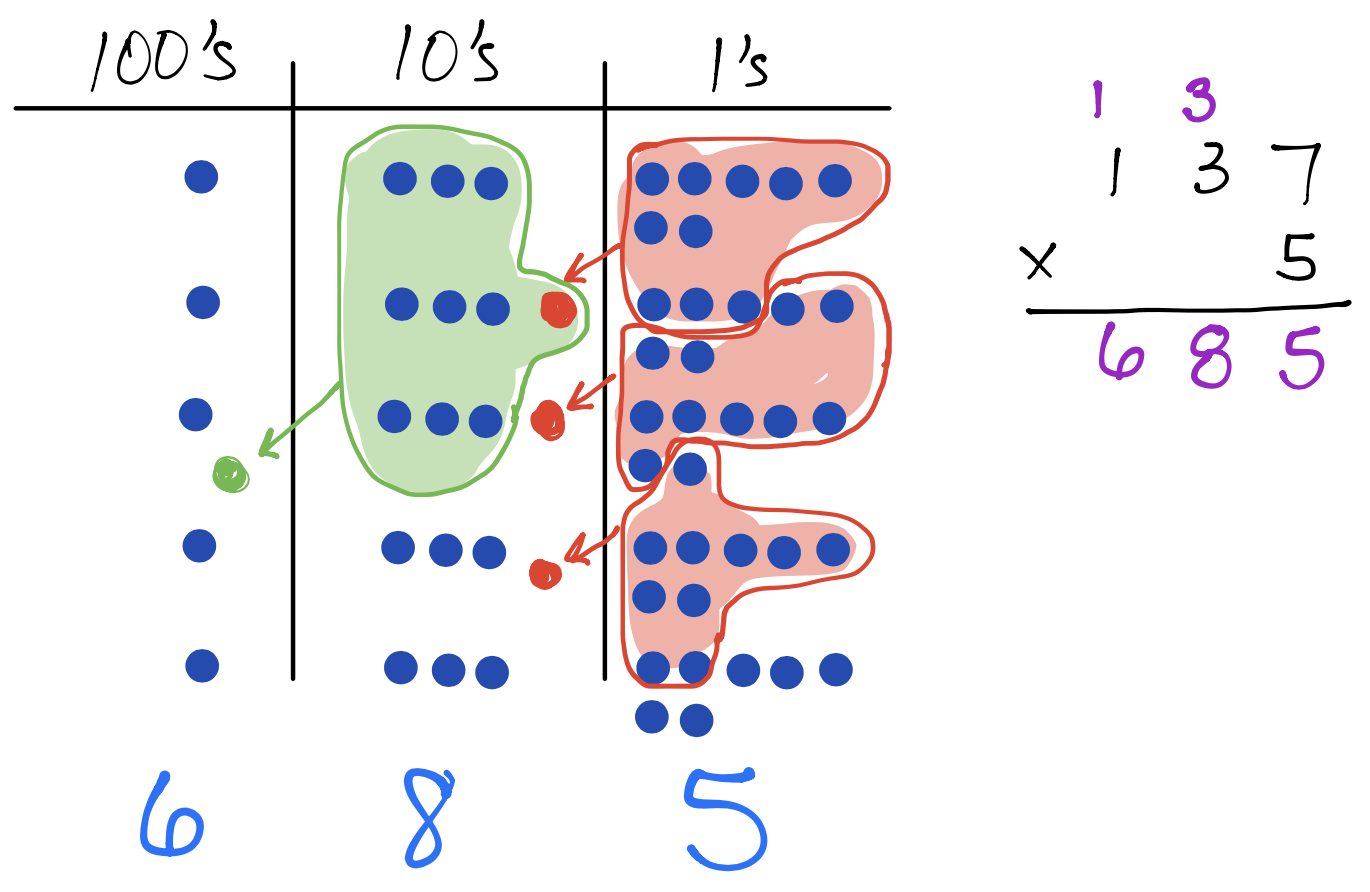For our final multiplication example, we will explore a possible technique for efficiently modeling the chips when there is a large number of groups we need to draw on the place value chart.

For example, 24 x 14 would require drawing either 24 groups of 14 or 14 groups of 24, both of which would be a lot of dots to draw!

 Let’s model 24 x 14 as 14 groups of 24, so we will begin by modeling 137 on the place value chart.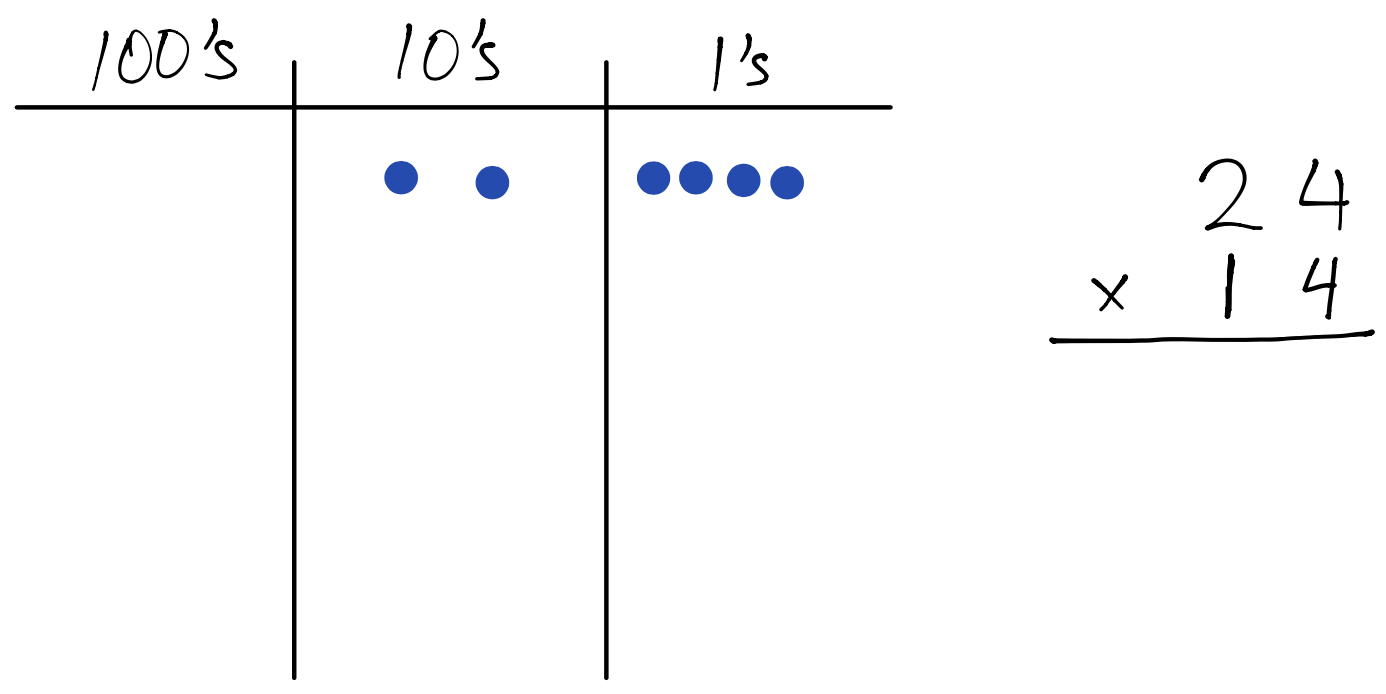To efficiently model 14 copies of a dot in any column of the place value chart, we use a line to represent 10 copies of that dot and then an additional 4 dots. We do this for each dot representing 24. At this point, we can see that the unsimplified product is 28 tens and 56 ones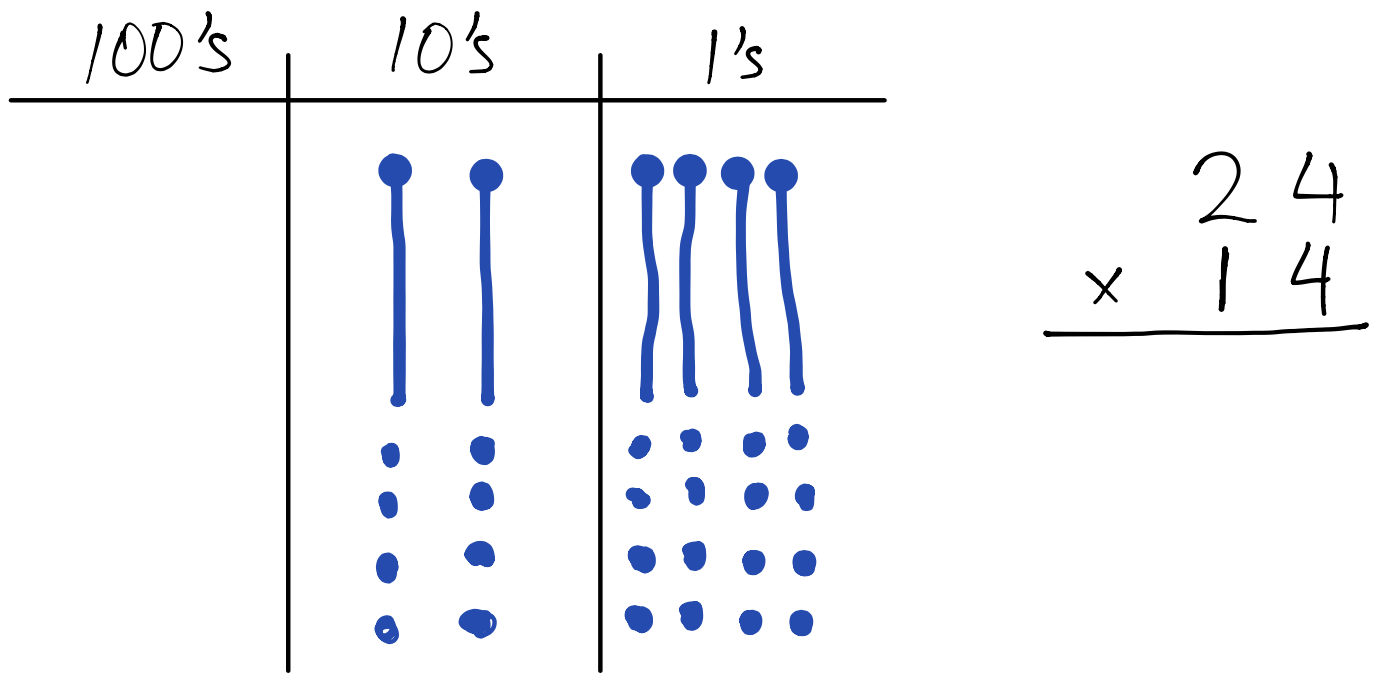Now we look for opportunities to do the exchange rate of 10 littles equals 1 big. Each line represents 10 dots, so we begin by exchanging each line (10 dots) with a dot in the column to the left. This is the same 10 littles equals 1 big that we have been doing in all the previous examples.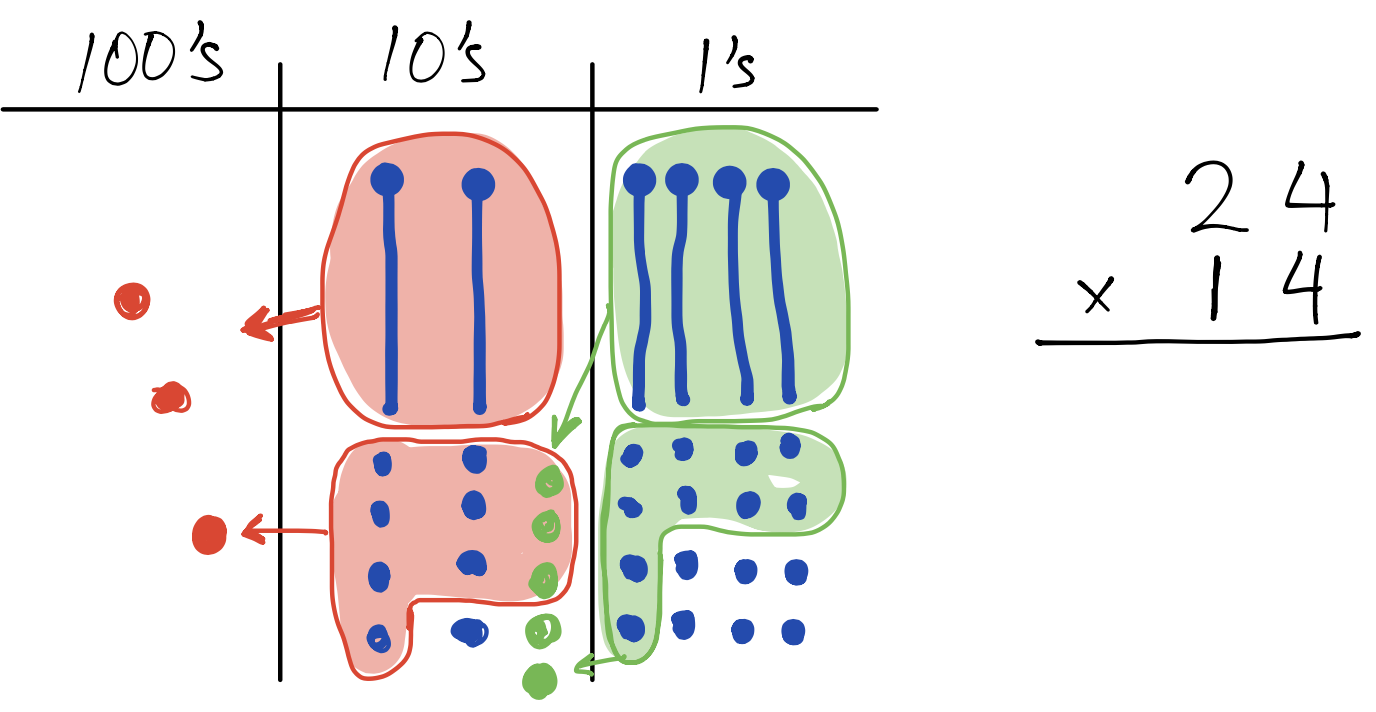After completing all the possible exchanges of 10 littles equals 1 big, we count the remaining dots in each column. 3 hundreds and 3 tens and 6 ones The product is 336.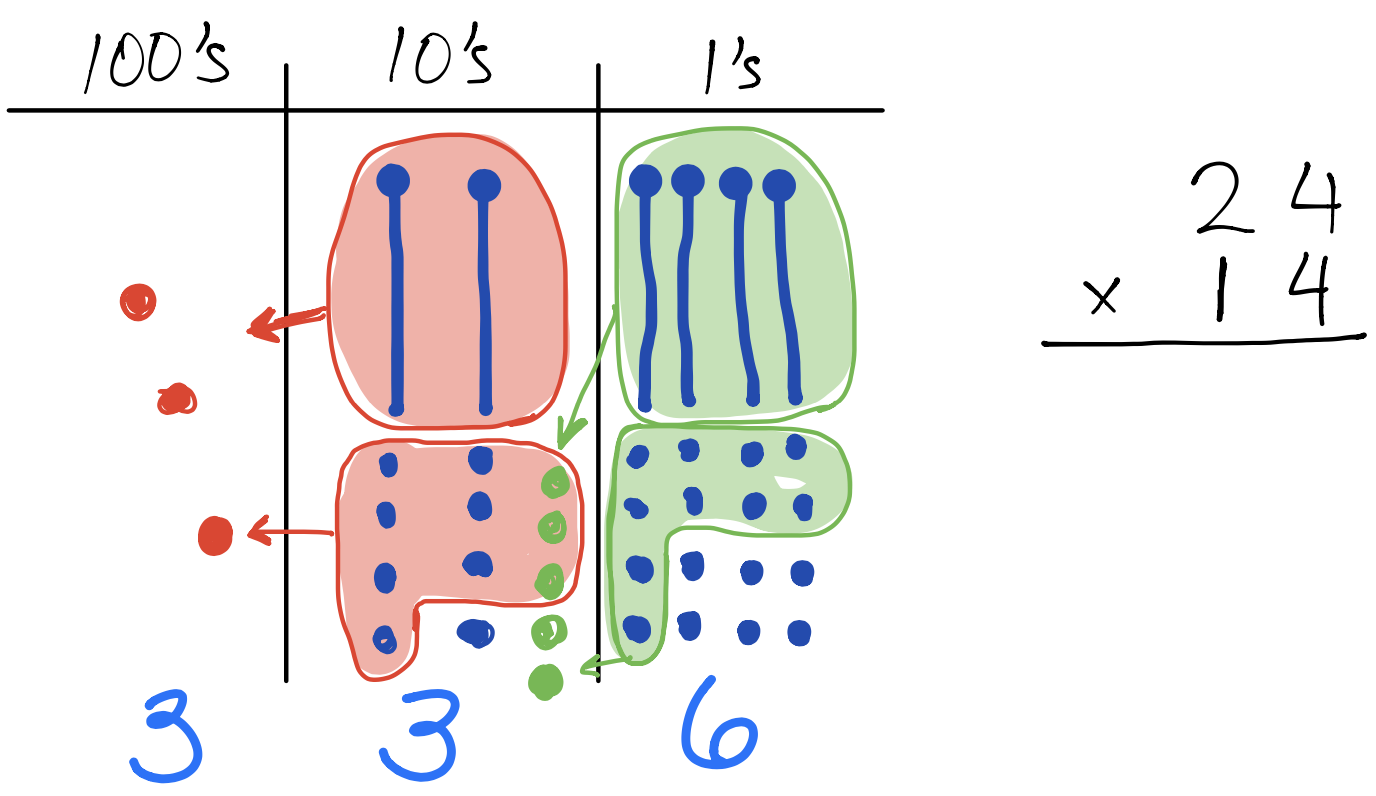The chip model can lead to the partial products method if we consider a different way to simplify all the lines and dots in the place value chart.

 Let’s revisit the visual model after representing 14 groups of 24.Now we consider the lines and dots in four parts.Let’s interpret the meaning and value of each of the four parts:         Upper left:         20 tens = 200         Upper right:         40 ones = 40         Lower left:         8 tens = 80         Lower right:         16 ones = 16 Then we add the four partial products to find the product of 336.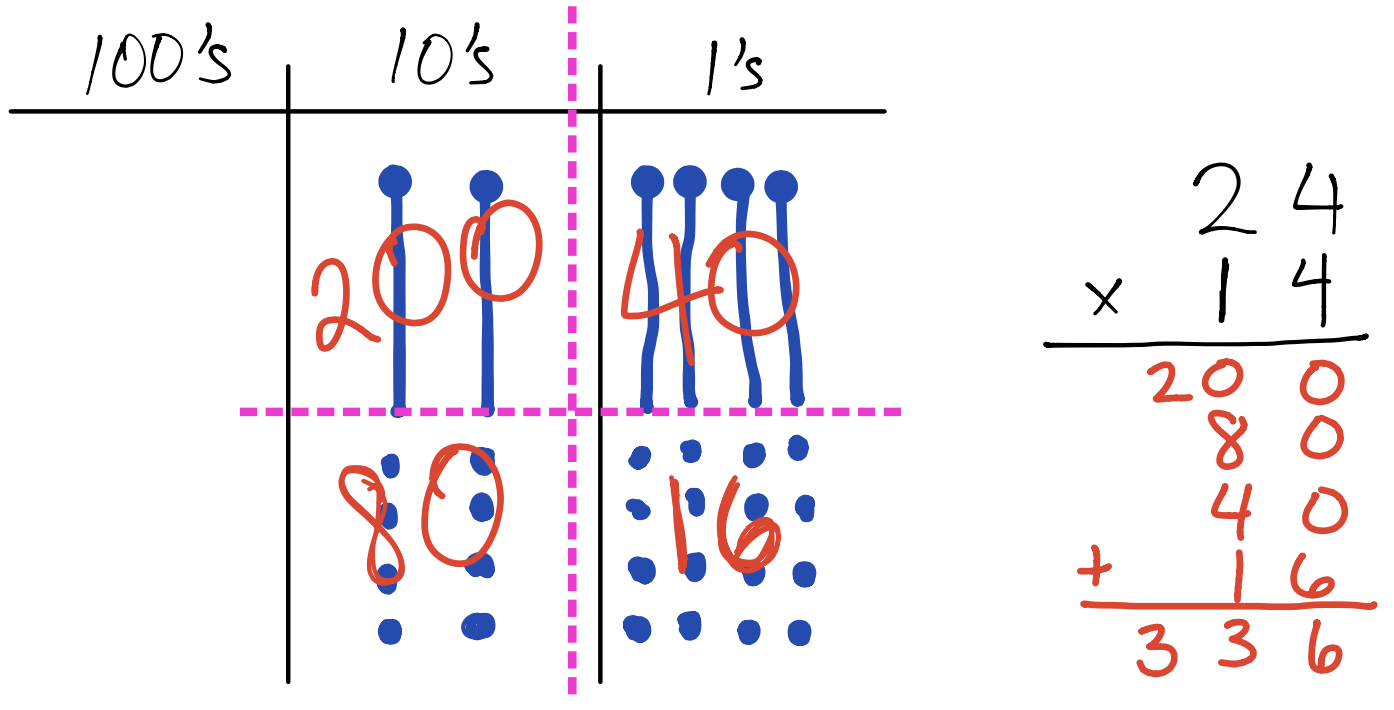To summarize this section on multiplication, there are two things for students to know:

1. Multiplication means equal groups
2. The exchange rate is 10 littles equals 1 big